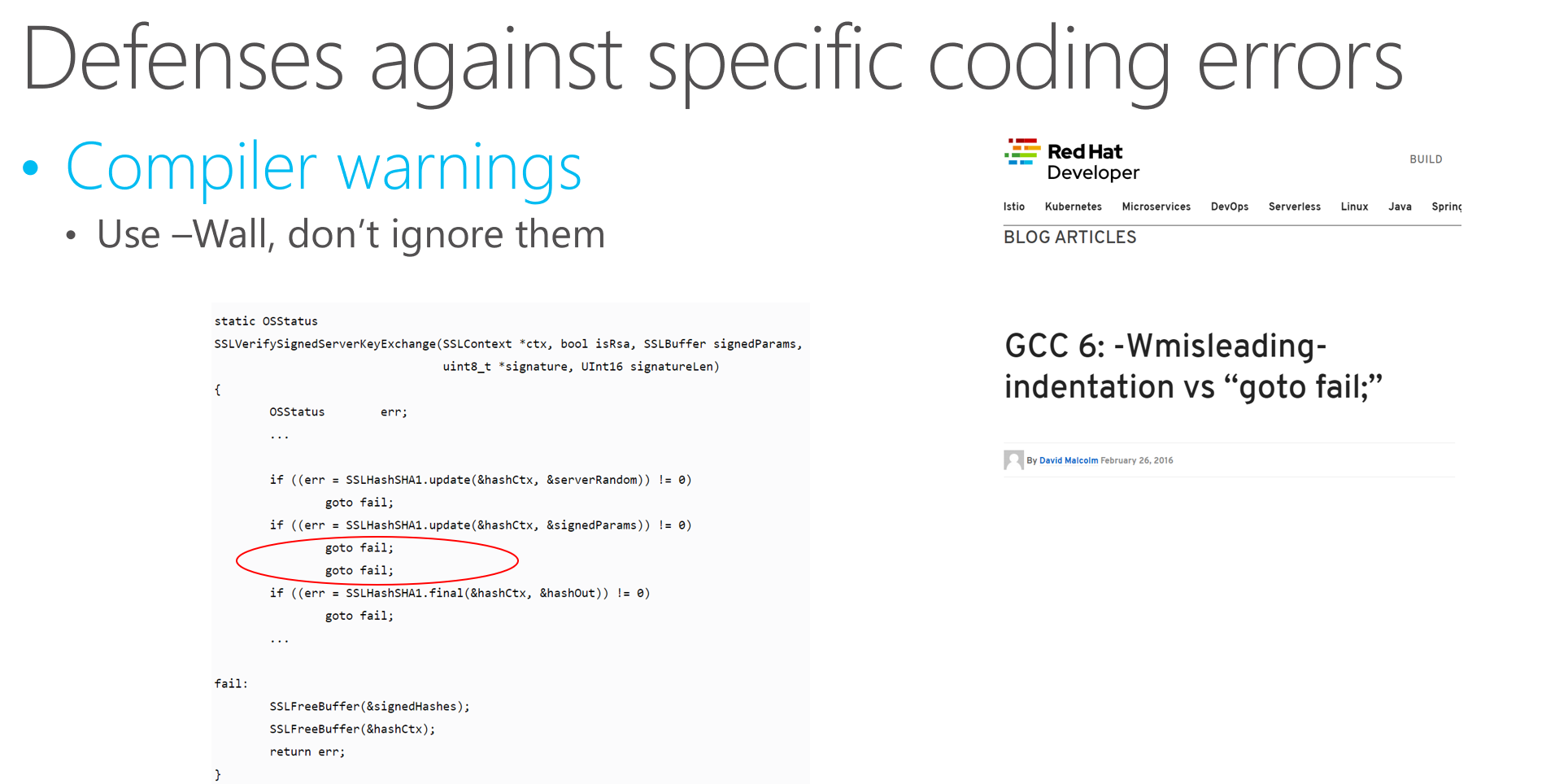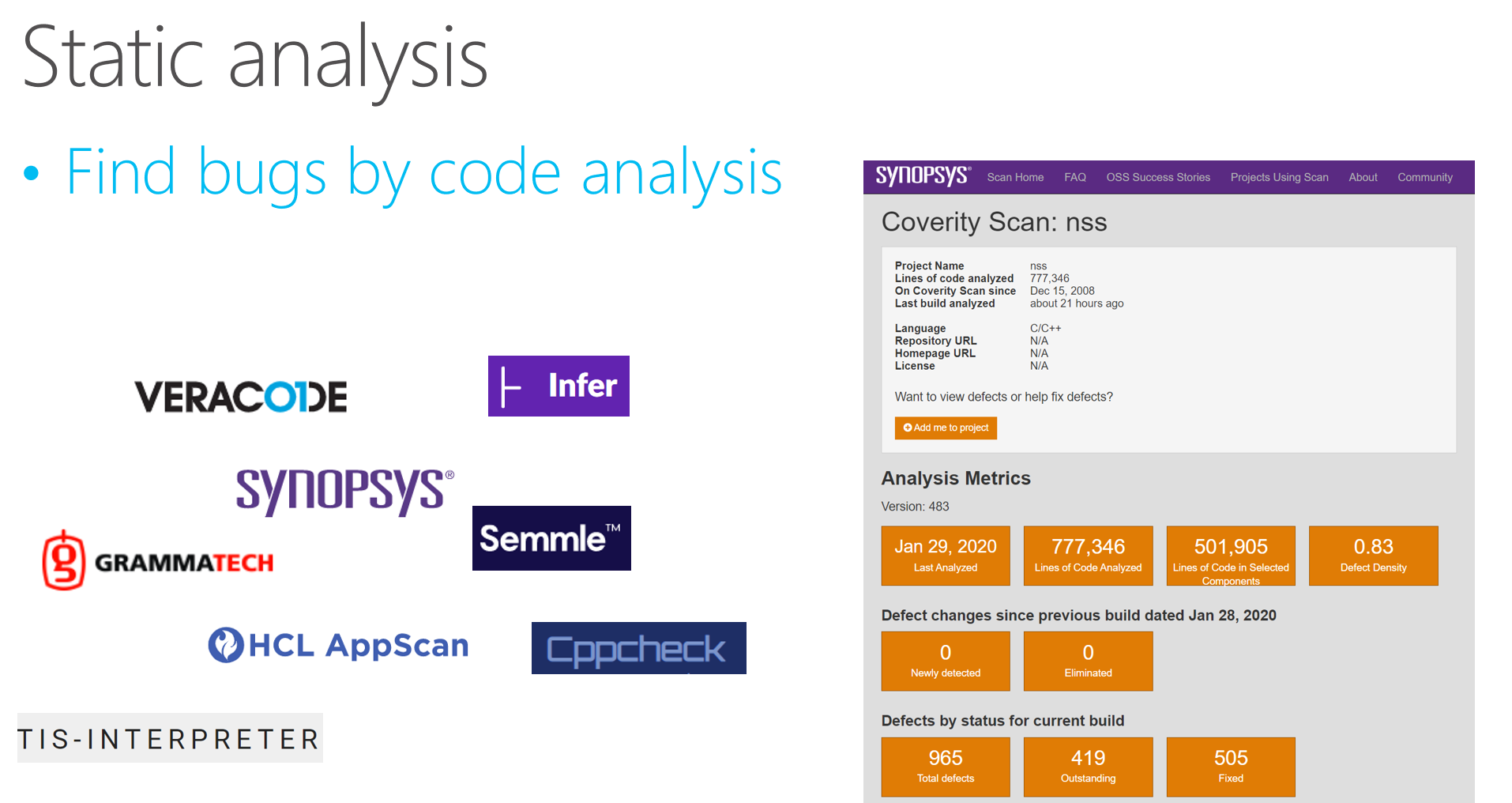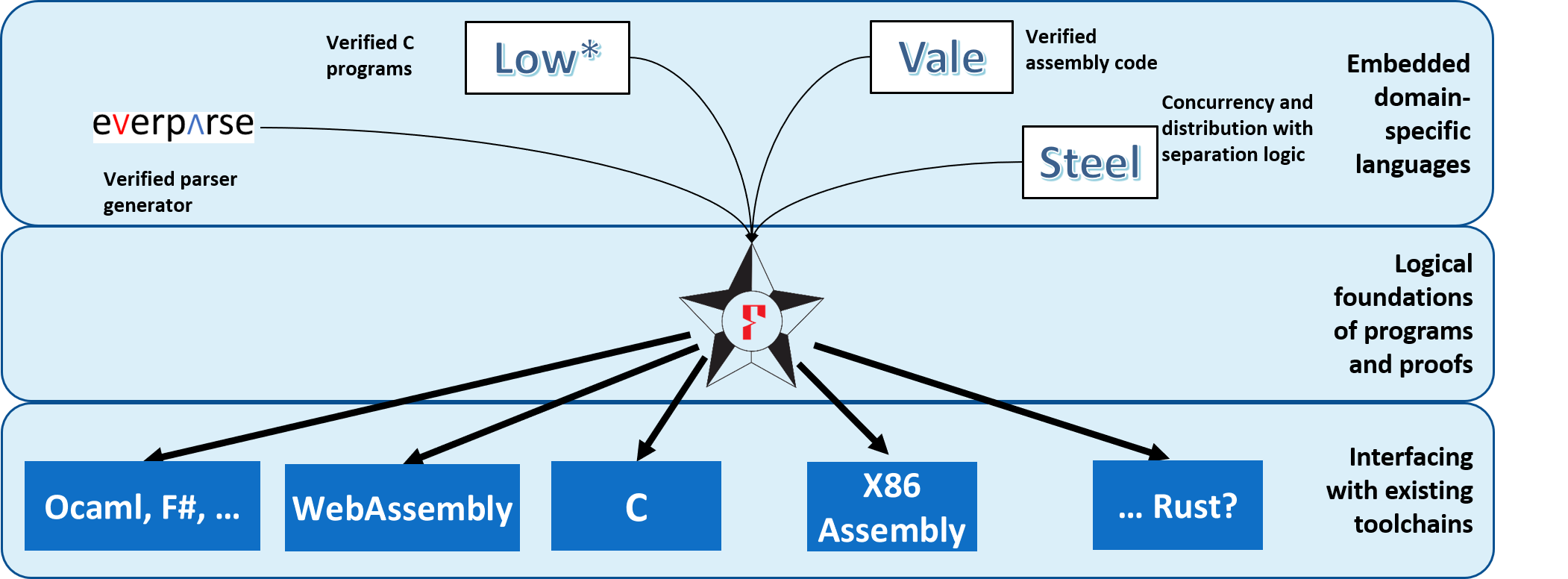## Proof-oriented Programming in F*

Microsoft Research

Oregon Programming Languages Summer School (OPLSS), 2021

## Embedding Proof-oriented Programming Languages in F*

Microsoft Research

Oregon Programming Languages Summer School (OPLSS), 2021

## Through Code Analysis (1)## Through Code Analysis (2)### Program Proofs at Scale

• Mathematical specifications of correctness and security

• Machine-checked proof that the code does not deviate from the spec

• Foundational: Against a formal machine model

• Integrated: Single theorem covering all the code

• Many successes in the past 10-15 years

• CompCert: A certified C compiler in Coq
• seL4: A verified micro-kernel in Isabelle
• Ironclad and Ironfleet: Verified distributed systems in Dafny

### Project Everest

• Building and deploying system components with proofs of correctness and security

• Focusing on secure communication software: TLS, QUIC, Signal, etc.

• But, also secure sub-systems, like measured boot, high-integrity key-value stores etc.

• Developed using the F* programming language

• Building and maintaining formal proofs at scale

• Multiple times a day, our continuous integration system verifies and builds more than 600,000 lines of F* code and proof

• Proof automation “in the small”

• 10^6 small proof obligations discharged by Z3 at each build

• Domain-specific languages with carefully designed full automation for specific kinds of proofs

• Modular abstractions to compose proven components

## Program Proofs in F* for Billions of Unsuspecting Users## Contributors (1)## Contributors (2)### Still expensive to develop proofs

• State of the art: 1 line of code : N lines of manual proof, where

• N=20, manual, interactive proof of trickiest code
• N=5, partially automated proofs of imperative code
• N=0.2, code generated by metaprograms
• N=0, very specific domains where proofs in specific DSLs can be fully automated
• Verifying a large piece of existing code, “after the fact”, is still too difficult

• Often writing a LOT more proof than code

• Pays to work in a framework optimized for proving and proof automation
• Pays to structure a program with its proof in mind

### Proof-oriented programming

• Programs and their proofs, co-developed

• Good synergies:

• Proofs can be simpler, because the program's structure is designed to facilitate it

• Programming can be simpler, since proofs guide program construction, e.g., unreachable cases can be ignored

• Programs can be more “daring”, since invariants help justify optimizations too risky to attempt otherwise

### F*: A Framework for Proof-oriented Programming

• Functional programming language with effects

• F* extracted to OCaml or F# by default
• Subset of F* compiled to efficient C code and to Wasm
• Semi-automated program verifier using automated theorem proving

• like Dafny, FramaC, Why3,
• With an expressive core language based on dependent type theory

• like Coq, Lean, Agda, Idris, Nuprl
• A metaprogramming and tactic framework for interactive proof and user-defined automation

• like Coq, Isabelle, Lean, PVS, etc.
• And many foundational program logics for embedded DSLs

• Many variants of Hoare logic for sequential programs
• Concurrent separation logic, for concurrent and distributed programs
• Relational Hoare logic, for program equivalence and security proofs

## Proof-oriented Programming Languages Embedded in F*### Outline of this course

• Lecture 1: Introducing F*

• Basic functional programming with dependent types
• Deep embeddings:
• Warm up: Simply typed lambda calculus
• Vale: A proof-oriented assembly language
• Lecture 2: Shallow embeddings of effectful languages

• Stateful programming with an ML-style heap
• Indexed computations and Dijkstra monads
• Security-oriented programming: An embedded language with information flow control

### Outline of this course

• Lecture 3: Layering effectful DSLs

• Low*: Programming and proving with a C-like memory model
• EverParse: A parser generator layered on Low*
• Lecture 4: Concurrent programming and separation logic

• Warm up: A total semantics for concurrency
• Deriving a concurrent separation logic for partial correctness
• Steel: A DSL for proof-oriented concurrent and distributed programming

### Basic types

• The empty type: It has no values

type empty =
• The singleton: It has exactly 1 value

type unit = ()
• Boolean: It has exactly 2 values

type bool = true | false

### Inductive type definitions

• Simple inductive data types
type list a =
| Nil : list a
| Cons : hd:a -> tl:list a -> list a
• But, actually, (mutually) inductive type families
type rbtree a : nat -> color -> Type =
| Leaf : rbtree a 1 Black
| R    : left:rbtree a h Black -> value:a -> right:rbtree a h Black -> rbtree a h Red
| B    : left:rbtree a h cl -> value:a -> right:rbtree a h cr -> rbtree a (h+1) Black

### Recursive functions

• Recursive functions

let rec factorial (n:int) : int =
if n = 0 then 1 else n * (factorial (n - 1))
• Inductive datatypes (immutable) and pattern matching

let rec map (f: a -> b) (x:list a) : list b =
match x with
| [] -> []
| hd :: tl -> f hd :: map f tl
• Lambdas (unnamed, first-class functions)

map (fun x -> x + 42) [1;2;3] ~> [43;44;45]

### Refinement types

type nat = x:int{x>=0}
• Informal mental model: A type describes a set of values

let empty = x:int { false } //one type for the empty set
let zero = x:int{ x = 0 } //the type containing one element 0
let pos = x:int { x > 0 } //the positive numbers
let neg = x:int { x < 0 } //the negative numbers
let even = x:int { x % 2 = 0 } //the even numbers
let odd = x:int { x % 2 = 1 } //the odd numbers
let prime = x:nat { forall n. x % n = 0 ==> n = 1 || n = x } //prime numbers
• Refinements introduced by type annotations (code unchanged)

let rec factorial (n:nat) : nat = if n = 0 then 1 else n * (factorial (n - 1))
• Logical obligations discharged by SMT (simplified)

n >= 0, n <> 0 |= n - 1 >= 0
n >= 0, n <> 0, factorial (n - 1) >= 0 |= n * (factorial (n - 1)) >= 0
• Refinements eliminated by subtyping: nat<:int

let i : int = factorial 42
let f : x:nat{x>0} -> int = factorial

### Dependent types

• Dependent function types (), here together with refinements:

val incr : x:int -> y:int{x < y}
let incr x = x + 1
• Can express pre- and post- conditions of pure functions

val incr : x:int -> y:int{y = x + 1}
• Exercise: Can you find other types for incr?

### Total, recursive functions

• Tot effect (default) = no side-effects, terminates on all inputs

let rec factorial (n:nat) : nat = (if n = 0 then 1 else n * (factorial (n - 1)))
• F* refuses to accept this type for factorial. Why?

val factorial : int -> int
  let rec factorial n = (if n = 0 then 1 else n * (factorial (n - 1)))
^^^^^
Subtyping check failed; expected type (x:int{x << n}); got type int

factorial (-1) loops! (int type in F* is unbounded)

### Semantic termination checking

• based on well-founded ordering on expressions (<<)

• naturals related by < (negative integers unrelated)
• inductives related by subterm ordering
• x << D x
• f x << D f
• lexicographic tuples %[a;b]
• %[a; b] << [a'; b'] if a << a', or a = a' and b << b'
• Derived from the subterm ordering using accessibility predicates
• arbitrary total expression as decreases metric

val ackermann: m:nat -> n:nat -> Tot nat (decreases %[m;n])
let rec ackermann (m n:nat)
: Tot nat (decreases %[m;n])
= if m=0 then n + 1
else if n = 0 then ackermann (m - 1) 1
else ackermann (m - 1) (ackermann m (n - 1))
• default metric is lex ordering of all (non-function) args

val ackermann: m:nat -> n:nat -> Tot nat

## Lemmas

let rec length (xs:list a) : nat =
match xs with
| [] -> 0
| _::tl -> 1 + length tl

let rec append (xs ys : list a) : list a =
match xs with
| [] -> ys
| x :: xs' -> x :: append xs' ys
• Prove that the length of append is the sum of the lengths of its arguments
let rec append_length (xs ys : list a) : Lemma (length (append xs ys) = length xs + length ys)
= match xs with
| [] -> ()
| x :: xs' -> append_length xs' ys
• Proof by induction on xs
• Base case, xs=[] is easy: append [] ys = ys /\ length [] = 0
• Step: Use IH by calling function recursively on smaller arguments
• Sugar: Lemma p = u:unit { p } 

## More Lemmas

let snoc l h = l @ [h]

let rec reverse (l:list a) : list a =
match l with
| [] -> []
| hd::tl -> snoc (reverse tl) hd
let rec rev_snoc (l:list a) (h:a)
: Lemma (reverse (snoc l h) == h::reverse l)
= match l with
| [] -> ()
| hd::tl -> rev_snoc tl h
let rec rev_involutive (l:list a)
: Lemma (reverse (reverse l) == l)
= match l with
| [] -> ()
| hd::tl -> rev_involutive tl; rev_snoc (reverse tl) hd

## Proof of a program: QUICKSORT

• Work through the online tutorial and you'll reach eventually a proof of correctness of quicksort.
let rec quicksort (f:total_order a) (l:list a)
: Tot (m:list a{sorted f m /\ is_permutation l m})
(decreases (length l))
= match l with
| [] -> []
| pivot::tl ->
let hi, lo = partition (f pivot) tl in
let m = quicksort f lo @ pivot :: quicksort f hi in
permutation_app_lemma pivot tl (quicksort f lo) (quicksort f hi);
m

### Demo: Two Deeply Embedded Languages

• Basics: factorial, lemmas, vectors

• Warm up: Simply Typed Lambda Calculus

• Vale: A Proof-oriented Assembly Language

## Lemmas, squashed types, and proof irrelevance

• Lemma p is sugar for Tot (u:unit{p})

• We write squash p instead of u:unit{p}

• So, Lemma p is sugar for Tot (squash p)
• The type squash p is a sub-singleton, i.e., it has at most one element ().

(** All proofs of [squash p] are equal  *)
val proof_irrelevance (p: Type) (x y: squash p) : Tot (squash (x == y))
• The type prop in F* is defined as all the subtypes of unit.

• i.e., For p:prop and proof of p, i.e., e:p is noninformative

### Equality

• Two notions of equality

• Definitional equality: if and only if .

• Provable equality: and are provably equal if is inhabited, i.e., you can build a term of the following type

type equals (#a: Type) (x: a) : a -> Type = | Refl : equals x x
• In F*, we write x == y to mean squash (equals x y).

### Extensionality: The Essence of F*

• Clearly, if x y then x == y.

• In intensional type theories, given e : t and t t', then by conversion e : t'.

• Equality reflection: In F*, like in other extensional type theories (e.g., Nuprl), if e:t and t == t', then e : t'.

• i.e., types conversion is possible through the silent use of provable equalities

• v: vec a (n + 0) is convertible with vec a n, since (n + 0) == n, although n + 0 n.

• However, this makes typechecking in F* undecidable

• Practically speaking, F* uses SMT to decide if/when a conversion is applicable

### Functional Extensionality, Subtyping and Eta reduction

• With equality reflection, it is possible to prove, when e == e':

(fun (x:a) -> e) == (fun (x:a) -> e')
• But, subtyping adds another level of subtletly (we got this wrong a couple of times)

• Due to refinement subtyping, we have (x:t0 -> t0') <: (x:t1 -> t1') when t1 <: t0 and t0' <: t1'.

• E.g., (int -> nat) <: (nat -> int).
• But, this means that eta reductions do not preserve types

• E.g., for f:int -> nat, reducing fun (x:nat) -> f x to f widens its domain.
• Given some f, g: int -> nat, proving equals (fun (x:nat) -> f x) (fun (x:nat) -> g x) does not imply equals f g (e.g., we may have f (-1) <> g (-1)).

• So, definitional equality in F* does not include eta reduction